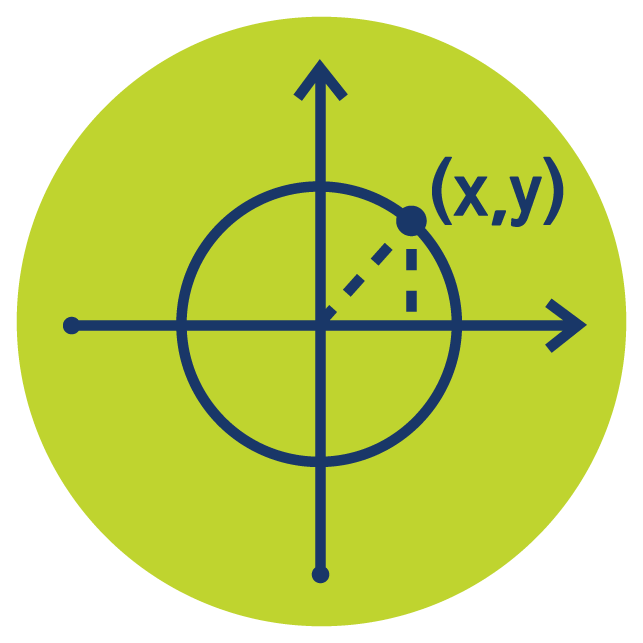Select Page# Trigonometry

The Trigonometry online course helps you develop skills which key to success across a range of Math courses. Trigonometry isn’t just about triangles but has many aspects and soon leaves the connection with triangles behind. Take this course and you’ll master trigonometry techniques through easy to follow instructional videos and practice question worksheets. View our classroom with this PREVIEW or learn more below and start your FREE one week Trigonometry TRIAL.

### Who is the Trigonometry Online Course For?

Trigonometry is a required core skill across many Math courses at all levels. The Trigonometry Online Course is especially useful for students who are new to trigonometry. However, experienced students can nonetheless refresh their knowledge or prepare for an assessment using our on-demand content. View the CLASSROOM.

### What is Taught in the Trigonometry Online Course?

Pythagoras’ Theorem, Trigonometric Ratios, Trigonometric Functions & Graphs, Reciprocal Trig Functions, Trigonometry with General Triangles, Radians & Exact Values, Trigonometric Identities, Basic Trigonometric Equations. You’ll soon master these topics with step by step videos and practice question worksheets – basically like having your own Trigonometry tutor 24/7. View the full Trigonometry online course SYLLABUS.

### What Will I Learn in This Course?

By taking the Trigonometry online course you will learn core and more advanced trigonometry skills which can then be used to solve a range of problem types now and subsequently. Since trigonometry appears in so many Math courses getting confident with these techniques now will certainly make all future Math courses easier. Students will gain experience of common trigonometry question types using our practice question worksheets with step by step solutions. Download FREE TRIGONOMETRY QUESTION WORKSHEETS.

### How Does Trigonometry Compare to Trigonometric Equations?

The Trigonometry online course covers many aspects of Trigonometry whereas Trigonometric Equations assumes most of these skills are already in place. Trigonometry is undoubtedly excellent foundation for Trigonometric Equations. Learn more about TRIGONOMETRIC EQUATIONS.

### What’s Next?

Trigonometry features in many Math courses so having these skills in place allows students to feel confident when they appear thereafter. Trigonometry is a key required skill in a range of Pre-Calculus & Calculus courses.

## Algebra to Pre-Calculus Course Bundle

Access all 6 courses in the bundle Algebra to Pre-Calculus to easily review older topics or jump ahead when ready.